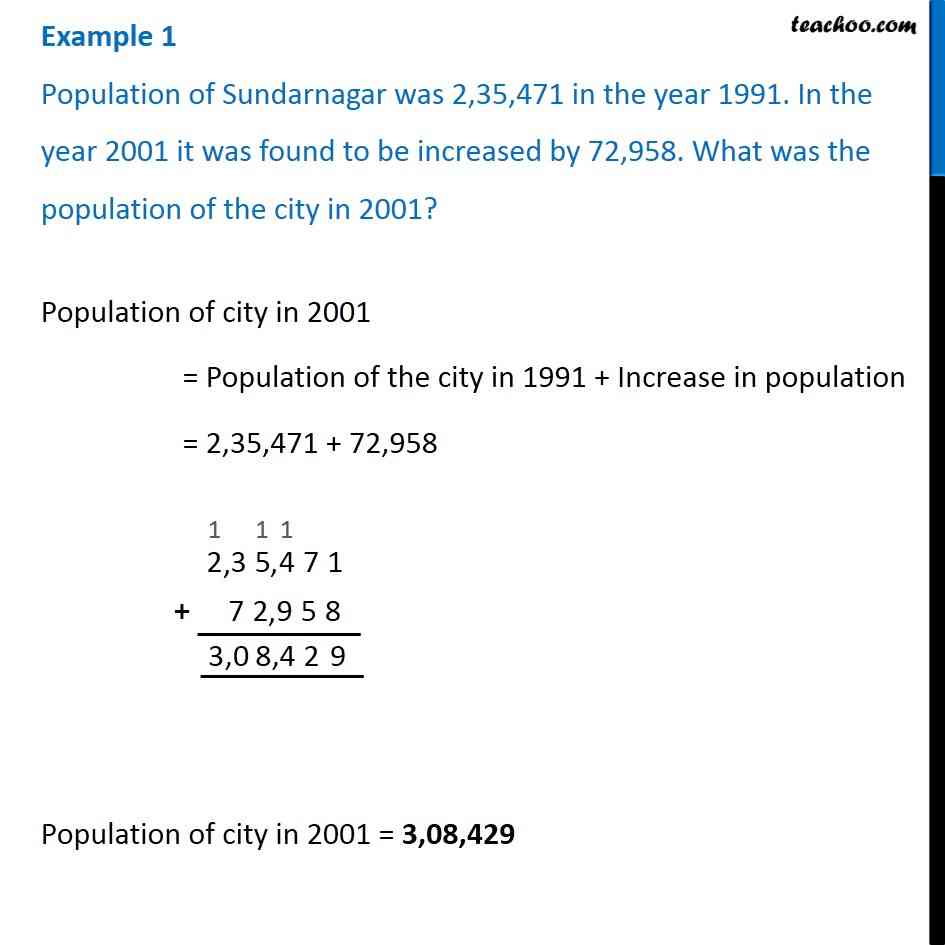1. Chapter 1 Class 6 Knowing our Numbers
2. Serial order wise
3. Examples

Transcript

Example 1 Population of Sundarnagar was 2,35,471 in the year 1991. In the year 2001 it was found to be increased by 72,958. What was the population of the city in 2001?Population of city in 2001 = Population of the city in 1991 + Increase in population = 2,35,471 + 72,958 Population of city in 2001 = 3,08,429 Example 1 Population of Sundarnagar was 2,35,471 in the year 1991. In the year 2001 it was found to be increased by 72,958. What was the population of the city in 2001?Population of city in 2001 = Population of the city in 1991 + Increase in population = 2,35,471 + 72,958 Population of city in 2001 = 3,08,429

Examples